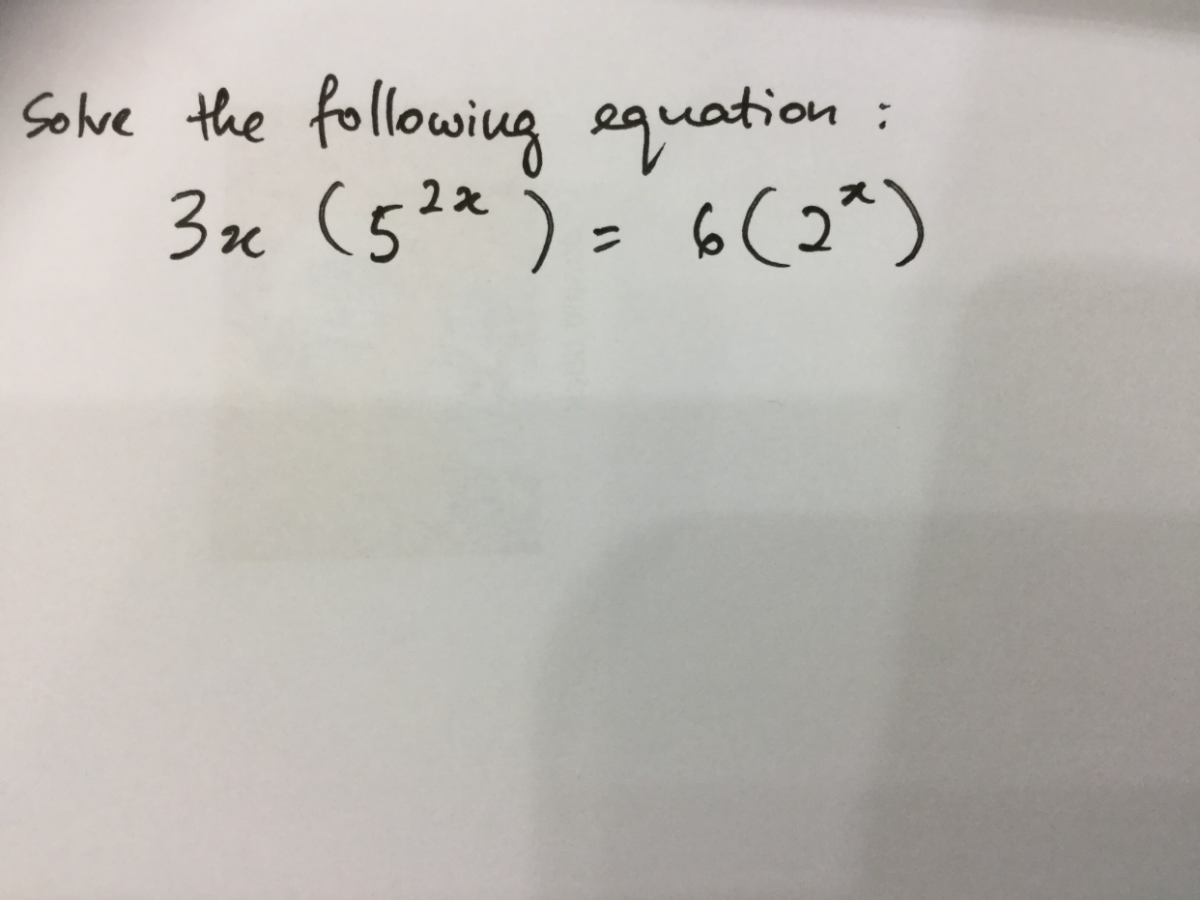# QuestionThank you so much Acestarling….for your great help!

Hi Jmaths.SG

The question is correctly written. Anyway, I have managed to solve the question after morning breakfast. Thank you for your great help too.

Hi Jmaths.SG

Hi TeaBoh,

By substituting x= 0.4944 into the equation shown in the pic, I get different values on LHS and RHS.

But if I sub x = 0.4944 into the equation suggested by Acestarling, then the values on LHS and RHS are the same.

From this, I can only conclude that the equation shown in the pic is wrong, and the error is “3x”, which should be “3^x”.

0 Replies 0 Likes

hi, TeaBoh

Sorry, I also not so confident how to solve this question.

If the question is 3x ( 52x ) = 6 ( 2x ) , then the answer is 0.4944.